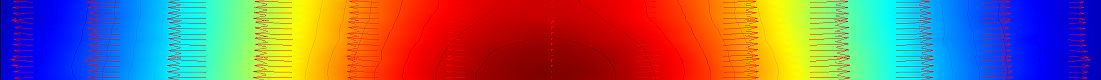# BME Differential Equations and Numerical Computations Research GroupThe members of our research group are researchers of the Department of Analysis and Operations Research of the Institute of Mathematics of BME. Our field of research is the modeling and simulation of processes that can be described by differential equations, or solving other tasks requiring computer modeling (Matlab, Python, Wolfram). The members have extensive experience in research and teaching in this area, which is demonstrated by numerous scientific articles, books and university lecture notes.

Members of our research group:

Our reseach areas:

Biomathematics, medical applications

• Epidemiological models and their qualitative properties
• Examination of models that can be described by delayed differential equations
• Cross-diffusion models for tumor growth and spread
• Active contour-based segmentation of aortic aneurysms
• Development and implementation of imaging algorithms
• Differential equations on networks (graphs).

Mechanical engineering, thermodynamics and fluid mechanics

• Solving heat conduction and diffusion problems using the finite difference and finite element methods
• Analysis of the qualitative reliability of continuous and numerical models (e.g. maximum principles, non-negativity)
• Realization of finite element simulations (e.g. dimensioning of support structures, modeling of the flow of polymers)
• Solving hydrological inverse problems
• Solving tasks using the operator splitting method
• Verification of the convergence of numerical procedures, Lax's equivalence theorem
• Eigenvalue problems, stability and distribution of eigenvalues, inverse eigenvalue problem and its stability

Chemistry

• Quantitative (numerical) and qualitative (symbolic) examination of differential equations of chemical reactions.
• Examination of the numerical methods of differential equations  (e.g. Runge-Kutta, linear multi-step methods)
• Estimation of parameters of models (differential equations) based on measurements.
• Chemical engineering applications
• Qualitative analysis and simulations of the stochastic models of reactions.
• Fuel cell models
• Estimation of fuel cell parameters

Image processing, image segmentation

Electrotechnics

• Finite difference and finite element solution of Maxwell's equations (use of FDTD and unconditionally stable schemes)
• Management of time-dependent material parameters during the operator splitting process
• Application of different boundary conditions (e.g. absorber or PML)

Our services:

• Construction of mathematical models for problems from different applications. Numerical solutions of mathematical models and visualization of the solution. Quantitative and qualitative analysis of continuous and numerical models
• Guidance on the applicability and properties of continuous and discrete mathematical models
• Guidance on the use of Matlab, Python and the Wolfram language (Mathematica).
• Presentation of possible mathematical and implementation tmethods in the form of mini-courses